# Chebyshev set

A setin a metric space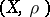such that for eachthere exists exactly one element of best approximation, that is, an element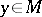such that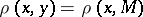. The existence and uniqueness of best approximating elements is a most simple condition, most convenient from the theoretical as well as from the practical point of view. This determines the role of Chebyshev sets in approximation theory and in the theory of Banach spaces. The notion of a Chebyshev set is a logical development of that of a Chebyshev system.

A finite-dimensional vector subspacewith basis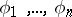is a Chebyshev set (Chebyshev subspace) if and only if the functionsform a Chebyshev system (equivalently, satisfy the Haar condition). Lines, planes, convex figures, and bodies in a Euclidean space are Chebyshev sets. Non-trivial examples of Chebyshev sets were first considered by P.L. Chebyshev . These were the subspace of algebraic polynomials of degree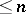and the set of rational functions with fixed degree of the numerator and the denominator in the space. A set in a Euclidean space is a Chebyshev set if and only if it is closed and convex.

In Lobachevskii geometry a Chebyshev set need not be convex . In a two-dimensional non-smooth normed space it is easy to construct a non-convex Chebyshev set. There exist non-smooth three-dimensional spaces in which every Chebyshev set is convex. The problem whether arbitrary Chebyshev sets in a Hilbert space are convex is unsolved (1987). At the same time there are proofs of the convexity of Chebyshev sets under supplementary conditions on the set and on the space, and conditions that are equivalent to convexity for Chebyshev sets (cf. Approximate compactness).

Since Chebyshev sets can be non-convex, one studies other characteristics of them. A Chebyshev setis called a sun  if for any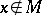and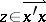(whereis the point ofnearest toandis the ray throughemanating from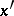), the pointis the point ofnearest to. For a Chebyshev setin a smooth space, the conditions "M is convex" and "M is a sun" are equivalent.

The properties of Chebyshev sets are closely related to approximative compactness and the continuity of a metric projection. Letbe a given Chebyshev set in a Banach space. If a)is a boundedly-compact set or b)is uniformly convex andis locally compact, thenis a sun (under the extra hypothesis "X is smooth" ,is a convex set). A Chebyshev set with a continuous metric projection in a smooth reflexive space is convex, and init is a sun. Every Chebyshev set in a uniformly-convex Banach space is connected (even its intersections with balls are connected). However, the set of functionsin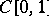, where,and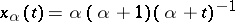for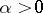, is a Chebyshev set with an isolated point, that is, it is not connected and it is not a sun . The question whether, in an arbitrary Banach space, every Chebyshev set that is a sun is connected, remains unsolved (1987).

In "nice" spaces there are quite a number of Chebyshev sets. In a Banach spaceevery convex closed set is a Chebyshev set if and only ifis strictly convex (strictly normed) and reflexive. In an arbitrary reflexive space there always exists a Chebyshev set, to wit a hyperplane. In an-dimensional Banach space there exist Chebyshev subspaces of all dimensions(cf. ). There exists a space in which there are no non-trivial Chebyshev subspaces. In a uniformly-convex Banach space, every closed subsetis an almost Chebyshev set, in the sense that the set of pointsfor which a unique nearest point inexists, is the complement of a meager set in. In a separable space there exist subspaces of all finite dimensions that are "almost Chebyshev sets" . There exists a space, isomorphic to a Hilbert space, in which the metric projection onto a certain Chebyshev subspace is discontinuous.

The notion of a Chebyshev set allows generalizations, for example, instead of the uniqueness of the element of best approximation one may require some kind of "regularity" of the set of elements of best approximation for each, such as compactness, connectedness or convexity. The results obtained for such generalizations are largely analogous to the corresponding results for Chebyshev sets.